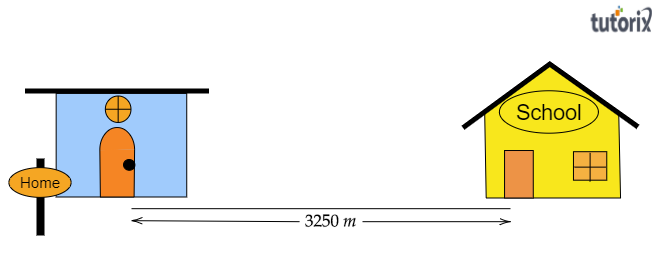# The distance between Radha's home and her school is 3250 m. Express this distance into km.

Given:

The distance between Radha's home and her school is $3250\ m$.

To do:

To express the given distance into $km$.

Solution:

Distance between Radha's home and her school$=3250\ m$

Meter and kilometer are two different units for measuring the length or distance. For measuring small distances meter is used and for measuring the larger distances kilometer is used.

Relation between $meter$ and $kilometer$ is as:

$\boxed{1\ km=1000\ m}$

Or $1000\ m=1\ km$

Or, $\boxed{1\ m=\frac{1}{1000}\ km}$

Let us convert the given distance$(3250\ m)$ into kilometers by using the above relation.

Therefore, $3250\ m=\frac{1}{1000}\times 3250\ km$

$=3.25\ km$Therefore, the distance between Radha's home and her school is $3.25\ km$.

Updated on: 10-Oct-2022

28 Views